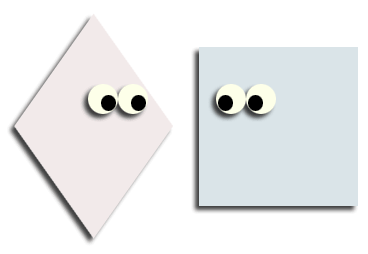### On rectangles and rhombiIllinois math instructor John Benson recently came across something that caught him by surprise in some elementary mathematics materials aimed at teachers.  He posted it to a listserv, which is where I came across it.

..There was a careful definition of rhombus followed by a statement that was well intentioned but that caught me by surprise.
"While most rhombi do not have right angles, a rhombus can have right angles"
This took me by surprise and I wanted to know what other knowledgeable and thinking math teachers from all grade levels thought about it, so I asked the question on the list serve, out of context.

I had several thoughts myself and wondered what others would think.  First, I think this is a great question to ask kids.  Many of them do not understand that a square is a rhombus ( using the inclusive definition, which is what I hope everyone uses all the time for everything, including
trapezoids) This raises the issue in an indirect way, which I think is a good thing.

I learned that I did not state the question well enough in that some of us thought that the angles formed by the diagonals are "angles of the rhombus" so the answer is all of them. I had not thought of that. That is why I put the question out there. I intended the question to be about the vertex angles. What a cool thing if some kid thought of that. When I use it with students, I shall leave the question as is and hope a student mentions the diagonal angles, congratulate that kid, and redirect the question to the one I intended.

So, there are infinitely many squares, and there are infinitely many non-square rhombi so the answer is not obvious. One line of thought is that the probability that a randomly selected angle would be 90 is zero so most are not squares. That raises the question of infinity, which is always an interesting place to go in a math class. I think it also raises the question of random. From where are these quadrilaterals being selected? If the angles are being randomly selected by a computer, then it depends totally on the level of precision the computer is using. If it is selecting an integer between zero and 180, then the likelihood is 1 out of 179, I think. If it is rounding to the nearest whatever, less likely. Are these not great questions for students to think about?

If this question is being asked to a third grader, I suspect the immediate response will be that squares are not rhombi, so none. Once convinced, then they will conclude that most are squares, because likely is about the ones that one encounters, and I suspect that the average person encounters way more squares that any other sort of rhombus.

So, one silly question can lead to so much interesting mathematics. The best part is that each of these strands will feed into something bigger and more important.